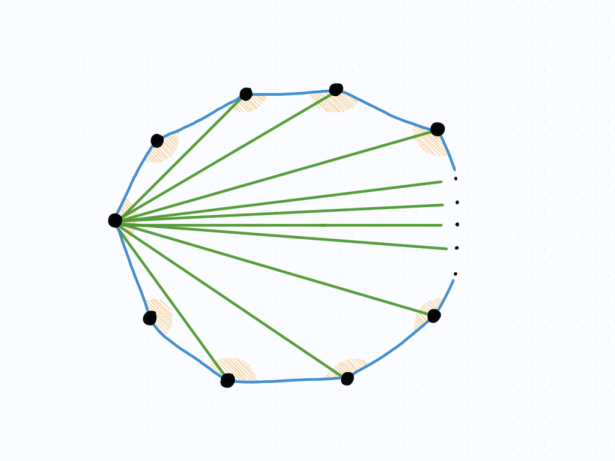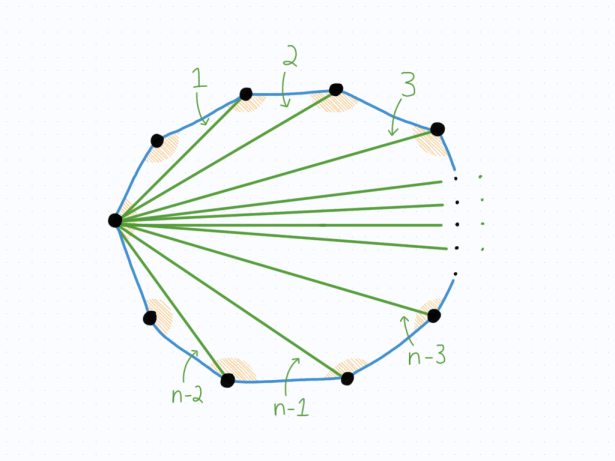# Interior Angles of a Regular Polygon

When I was in secondary school, I was given a table listing the various properties of polygons. It had a column for the number of sides the shape had, the name of the shape, the value of one interior angle, and the sum of the interior angles. This was a chart that most of us copied onto our memory aid, and we didn’t think about it more than that.

We were also presented with an equation for calculating the interior angle of a regular polygon with n sides. It looked like this:

Interior angle = (n-2)×180°/n.

Where I went to school, we weren’t presented with a proof of this result (at least, not from what I can remember). Instead, we were merely told them as facts to copy down. Honestly, I used this result so little that I never cared to try and figure out the meaning behind it. But when I look at it now, the question that jumps out to me is: Where does the (n-2) term come from?

I was recently motivated to answer this question for myself as I needed to explain it to a student. If there’s a lesson here, it’s that I find teaching an idea to be much more satisfying when I don’t have to appeal to the boring argument of “mathematics just works”. So, I want to go through the thought process of someone trying to come up with this result. It’s a lovely argument of geometry, and I think you will appreciate its visual nature.

To start with, let’s get rid of the degrees and go to the more natural radians. As such, 180° will become π, like it should be.

Next, to answer this question, we need to have a regular polygon. The argument shouldn’t be any less clear if we jump straight to the generalization, so we will take a polygon with n sides. It might look something like this:Our goal is to calculate the value of one interior angle. However, since our polygon is regular (meaning the side lengths and angles are all the same), we can just focus on finding the sum of the interior angles. Then, we know that the sum can be divided by n to find the value of one interior angle.

To get a visual, we want to calculate the sum of the following angles:Here’s the cool part. Let’s start at one vertex, say the one on the left. Then, what we can do is connect this vertex to all of the other vertices.This creates a bunch of triangles. How many? Well, notice that each time a new connection is made, we get a new triangle. Therefore, the question becomes: how many connections are there?

We want our left vertex to be connected to all of the others. However, the two vertices which are directly adjacent to the left vertex don’t get a new connection to them, while every other one does. As such, the total number of connections (and hence, triangles) is (n-2).The final thing to notice is that the angles within all of the triangles are precisely what we want. In other words, if you add up all of the angles inside the various triangles, they exactly form the sum of interior angles of our polygons.

Plus, we know the sum of the angles in any given triangle! It’s simply π. Therefore, we just have to multiply the number of triangles by the sum of angles in a triangle, which gives us (n-2)π.

And there we go! To find the value for a single interior angle, we need to divide the above result by n. This reproduces the values that the table I had in secondary school showed.

What I find tragic about this is that it’s such a simple geometrical argument, and yet we don’t show this to students. I’m just finding it out now, years after I graduated from secondary school. I think this is a powerful argument for how “simple” results can still be the source of interesting mathematics. You don’t have to climb the ladder of abstraction to dizzying heights in order to find something interesting. Even a simple question about polygons can lead to a fascinating analysis.

Therefore, it’s worth taking the time to brush up on old results that you have learned in the past and make sure you have good arguments for them.